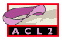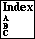## DEFTHEORY

define a theory (to enable or disable a set of rules)
```Major Section:  EVENTS
```

```Example:
(deftheory interp-theory
(set-difference-theories
(universal-theory :here)
(universal-theory 'interp-section)))

General Form:
(deftheory name term :doc doc-string)
```
where `name` is a new symbolic name (see name), `term` is a term that when evaluated will produce a theory (see theories), and `doc-string` is an optional documentation string (see doc-string). Except for the variable `world`, `term` must contain no free variables. `Term` is evaluated with `world` bound to the current world (see world) and the resulting theory is then converted to a runic theory (see theories) and associated with `name`. Henceforth, this runic theory is returned as the value of the theory expression `(theory name)`.

The value returned is the length of the resulting theory. For example, in the following, the theory associated with `'FOO` has 54 runes:

```ACL2 !>(deftheory foo (union-theories '(binary-append)
(theory 'minimal-theory)))

Summary
Form:  ( DEFTHEORY FOO ...)
Rules: NIL
Warnings:  None
Time:  0.00 seconds (prove: 0.00, print: 0.00, other: 0.00)
54
ACL2 !>
```